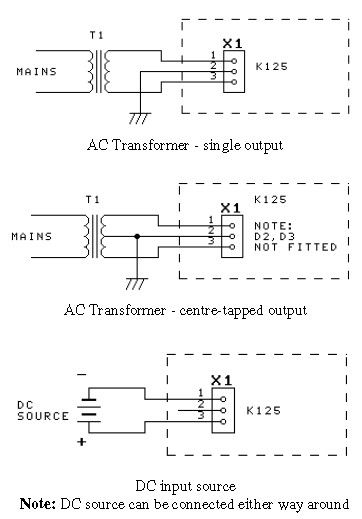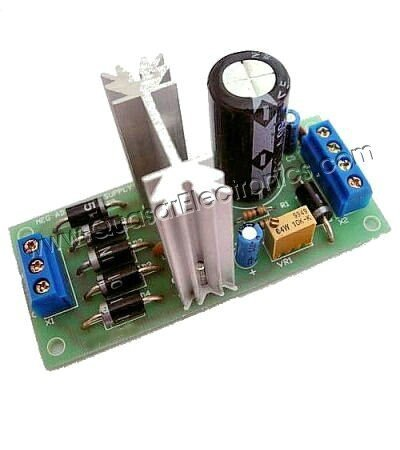# 1.5A Negative Adjustable Power SupplyThis is the circuit diagram of negative adjustable power supply based LM337T. This circuit can be used for devices which required negative supply to work. The regulator LM337T is capable of supplying 1.5A over an input voltage range of 1.2V to 37V. They need at least a 2.5V input-output voltage differential. In practice the limiting factor on the output voltage & current will be the power dissipated by the regulator. For example, if the input voltage is 30V & the output voltage is 10V and the output current is 1A then the power dissipated by the heatsink is (30-10)*1 or 20W. This would need a big heatsink. So it is desirable to keep the input voltage as low as possible to achieve the required output.

LM337T Features:

• Output-Current in Excess of 1.5 A
• Output-Adjustable Between -1.25 V and -37 V
• Internal Thermal Overload Protection
• Internal Short-Circuit Current Limiting
• Output-Transistor Safe Area Compensation
• Floating Operation for High-Voltage Applications

Components List:

Resistors (0.25W, 5%)
R1 = 330
R2 = 1K
VR1 = 10K 10-turn trimpot

Capacitors
C2,4 = 100nF ceramic
C12 = 200uF 50V electrolytic
C3,5 = 10uF 63V electrolytic

Semiconductors
D1-6 = 1N5403
REG = LM337T

How The Circuit Work:

Diodes D1-4 form a bridge rectifier which converts the AC input voltage into a DC level. They also allow a DC input voltage to be connected either way around.

Capacitor C1 smooths the DC output of the bridge whilst C2 provides high frequency decoupling. The LM337T is an adjustable regulator IC providing the desired output voltage. Diode D5 is reversed biased during normal operation and is used to protect the regulator if the output is connected to a voltage of the same polarity (eg battery). Diode D6 protects the regulator if a reverse polarity voltage is connected to the output.

The regulator develops a nominal 1.25V reference voltage between the output and adjust terminals. This constant voltage is applied across R1, causing a constant current to flow. This constant current flows through trimpot VR1. By varying VR1, the voltage across it will vary and hence the output voltage can be set.

Input Configuration (Connection to the Transformer):

There are a number of different input configurations that can be used with this kit. The configuration chosen will depend on the available voltage source. Wherever possible, keep the input voltage as low as possible to achieve the required output. This minimises the heat dissipation on the regulator.Kit Version of 1.5A Negative Adjustable Power Supply Circuit:Downloads:

Power supply is an electronic device that supplies electric energy to an electrical load. The primary function of a power supply is to convert one form of electrical energy to another and, as a result, power supplies are sometimes referred to as electric power converters. Some power supplies are discrete, stand-alone devices, whereas others are built into larger devices along with their loads. Examples of the latter include power supplies found in desktop computers and consumer electronics devices.

## Related Post "1.5A Negative Adjustable Power Supply"

Comments are closed.